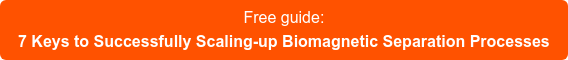Blog

Stablishing a separation time is the most usual way to specify a biomagnetic separation process. The problem is that this magnitude is not only related with the magnetic beads suspension, but also with the specific system used to perform the separation.Notice that the separation time would depend on the distance travelled by the beads and its speed. Assuming a constant speed, the bigger the vessel, the larger the separation time would be. Then to keep separation time constant, the force should be higher, something not easy to achieve at a reasonable cost. Even if it would make economic sense to build the system with higher force for larger volume, using a higher magnetic force would imply a higher risk of irreversible aggregation and the separation conditions would be very different that the verified ones at small volumes. As consequence, is important to define the separation time for a given process, but trying to keep it constant at every scale is misleading. Separation time is a consequence of the applied magnetic force, that should be the parameter defining the behavior of the beads and avoid losses and/or irreversible aggregation problems.The speed of the magnetic beads depends on the competition between the applied magnetic force and the drag force. The first would depend on the interaction between the magnetic moment of the beads and the profile of the applied magnetic field (remember you need an inhomogeneous magnetic field to have a force). The second depends on the viscosity of the buffer and the beads diameter.

For a given suspension (specific magnetic beads, at a given concentration in a defined buffer), we will need a constant magnetic force to have constant speed. Using classical magnetic separators, the force decrease with the distance, the beads closest to the magnet would move faster (higher force) than the beads farthest (lower force). Then, the separation time would be defined by the farthest beads. The bigger the vessel, thus the distance to the farthest beads. Still worst, as force decrease fast with the distance, the initial speed (force) would be lower as bigger is the vessel. For this reason, the separation time scales exponentially when the scale up is done using classical separators.

If we can generate a constant magnetic force, as advanced biomagnetic separation systems do, the separation time would scale proportionally to the distance travelled by the beads. That means it would be proportional to the diameter of the bottle is a cylindrical flask is used. For example, using the same magnetic force, the separation time using a 1 L borosilicate bottle (101 mm) would be 2.8 times the separation time using a 100 ml bottles (36 mm), even if the volume would be 10 times. Scaling from 100 ml to 10 L (227 mm) would imply to increase production volume by a factor 100 with a separation time 6.3 larger.

As the biomagnetic separation time is not the longer step on the process (for large volumes, pumping out the supernatant, adding the clean buffer and re-suspending the beads are processes taking much longer than the few minutes of the separation), the magnetic force can be lower at larger volume systems. That helps to keep weight (and costs) of advanced biomagnetic separation systems. For this reason, when the plan is scaling up the process it is advisable not to

use the maximal magnetic force available at small scale, but to replicate the conditions of the force available (at a reasonable cost) at very large volume.

Related news: